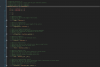# Asset - Scripts[VECTOR2] xVector2 is yet a another clean vector implementation (FREE)

#### xDGameStudios

##### Member

xVector2 (marketplace)

xVector2 (itch.io)

[API IMAGES][INFORMATION]

This is a Vector2 Class asset libary with a good amount of functionality to get you covered for most tasks (GMS 2.3+)

[QUICK GUIDE]

1) This module implements a constructor class so you just need to do:

GML:
``````var _vector = new Vector2(10, 10); // This creates a x: 10, y: 10 vector
var _newVector = _vector.multiplyBy(2); // This multiplies "vector" by 2

// NOTE: methods don't mutate the current instance. Meaning a new vector is created every time.
// Keeping the original vector unchanged.``````
2) However you may want to change the original vector. For your convenience I've included a Vector2Utils class:

GML:
``````var _vector = new Vector2(3, 7);

Vector2Utils.normalize(_vector); // This Vector2Utils "static" struct mutates the original vector.

// NOTE: This allows every-step computations to keep a lighter impact on the GC.``````
[MANUAL]

This library provides a set of useful function that you can use to create and manipulate Vector2:

GML:
``````var _vector = new Vector2(10, 10);

_vector.length() // returns the length of the vector

_vector.legnthSquared() // returns the squared length of the vector

_vector.withMagnitude(magnitude) // returns a new vector with the given magnitude.

_vector.normalize() // return a new normalized vector.

_vector.reflect(normal) // returns a new reflected vector according to a normal vector.

_vector.limit(min, max) // returns a new vector with clamped x/y components.

_vector.interpolate(dest, amount) // return a new vector "lerped" towards the destination by a given amount.

_vector.add(vector) // returns a new vector with the sum result.

_vector.subtract(vector) // returns a new vector with the subtraction result.

_vector.multiply(vector) // returns a new vector with the multiplication result.

_vector.multiplyBy(amount) // returns a new vector multiplied by a single value.

_vector.divide(vector) // returns a new vector with the division result.

_vector.divideBy(amount) // returns a new vector divided by a single value.

_vector.negate(); // returns a new negated vector (-x, -y).

_vector.equals(vector) // performs a equality (value) check between two vector2 instances.

_vector.copyToArray(array, index) // stores the vector elements in a given array starting at the given index.``````
Regarding the mutable functions inside the Vector2Utils namespace the functionality is very much the same. Please check the fully documented source code.

[COMPATIBILITY]

The asset is fully compatible with GMS2.3+ and is purely written in GML making it compatible with all exports available.

Last edited:
•RefresherTowel

#### CanalWeasel

##### Member
This looks really nice, thank you!

•xDGameStudios

#### xDGameStudios

##### Member
Thank you so much just released an Update to it:

Version 1.0.3
Change log: Fixed an issue regarding a missing comma.